# Bond Dissociation Energies (for A-B Bond broken AH, kJ/mol Bond broken → A A4, kJ/mol +...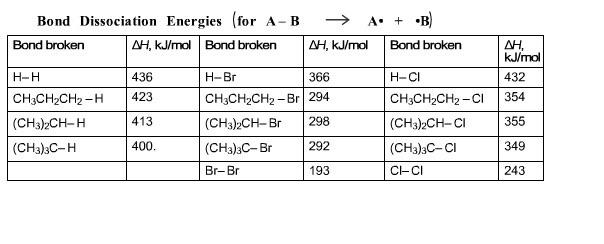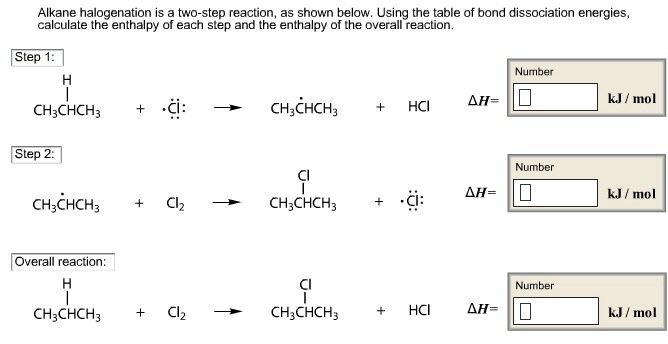Bond Dissociation Energies (for A-B Bond broken AH, kJ/mol Bond broken → A A4, kJ/mol + B) Bond broken mo H-H CH3CH2CH2-H (CH3)2CH-H (CH3)3C-H 436 423 413 400. H-Br 366 CH3CH2CH2-Br 294 (CH3)2CH-Br 298 (CH3)3C-Br292 Br-Br 193 H-CI 432 CH3CH2CH2-CI 354 (CH3)2CH-CI 355 (CH3)3C-CI 349 CI-CI 243
Alkane halogenation is a two-step reaction, as shown below. Using the table of bond dissociation energies, calculate the enthalpy of each step and the enthalpy of the overall reaction. Step 1: Number CH3CHCH3 + c → CH3CHCH3 + HCI AH= O kJ/mol Step 2: Number CH?CHCH3 + Cl2 → CH3CHCHI + “: AH-O J/mol Overall reaction: Number Hách, , , CH, CÁCH, + HOI A - B CH3CHCH3 + Cl2 → CH3CHCH3 + HCI AH= ] / mol kJ/mol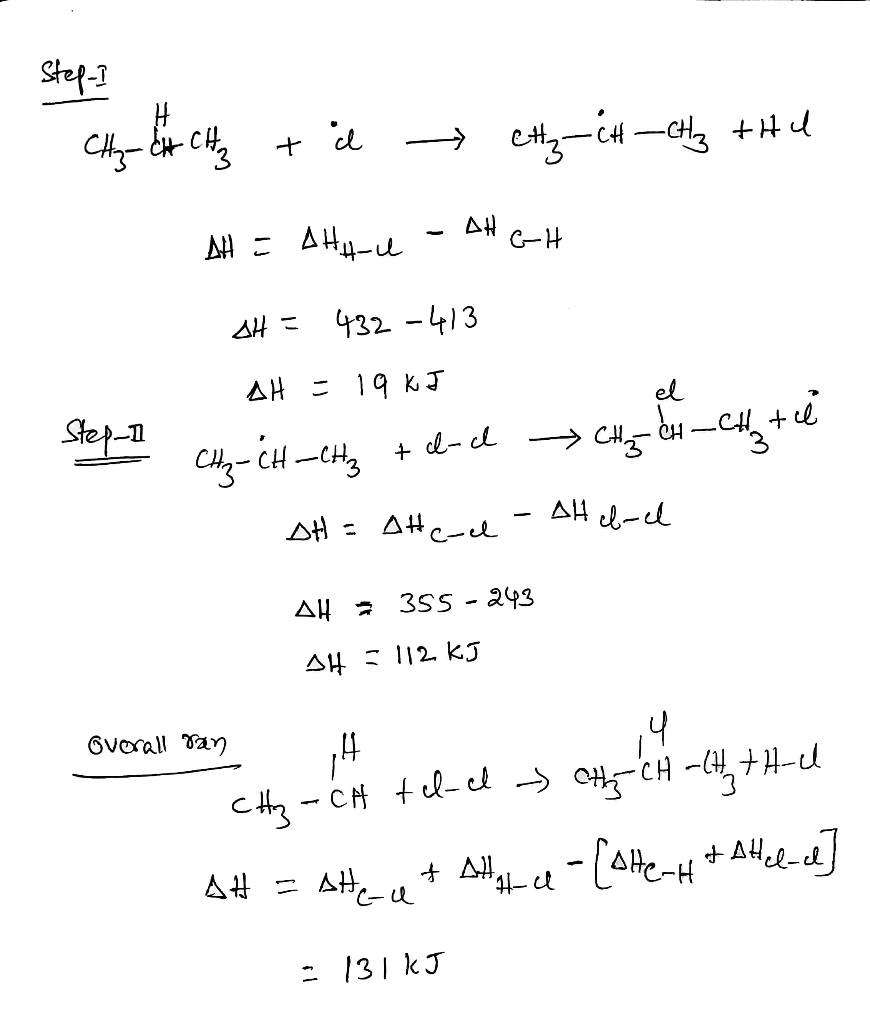#### Earn Coin

Coins can be redeemed for fabulous gifts.

Similar Homework Help Questions
• ### C-H bond BDE (kJ/mol) CH3-H 439 C2H:-H 422 (CH3)2 CH-H 414 (CH3)3 C-H 405 Table of...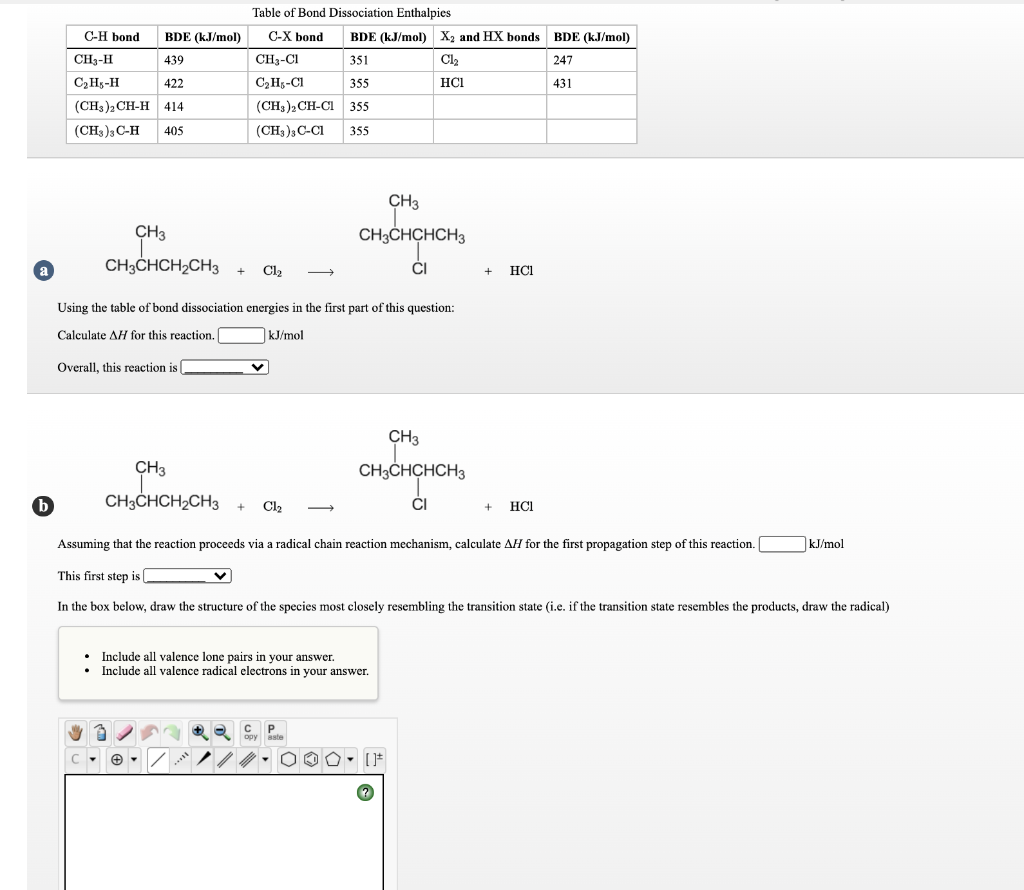C-H bond BDE (kJ/mol) CH3-H 439 C2H:-H 422 (CH3)2 CH-H 414 (CH3)3 C-H 405 Table of Bond Dissociation Enthalpies C-X bond BDE (kJ/mol) X2 and HX bonds BDE (kJ/mol) CH3-C1 351 CI 247 CH-C1 355 НСІ 431 (CH3)2CH-C 355 (CH3)3C-CI 355 CH3 CH3 CH3CHCHCH3 CH3CHCH2CH3 + a Cl2 CI HCI Using the table of bond dissociation energies in the first part of this question: Calculate AH for this reaction. kJ/mol Overall, this reaction is CH3 CH3CHCHCH3 CH3 CH3CHCH2CH3 + b...

• ### 1. Bond Dissociation Energies and Free Radicals Bond Bond Dissociation Energies (kJ/mol) Bond BDE Bond BDE...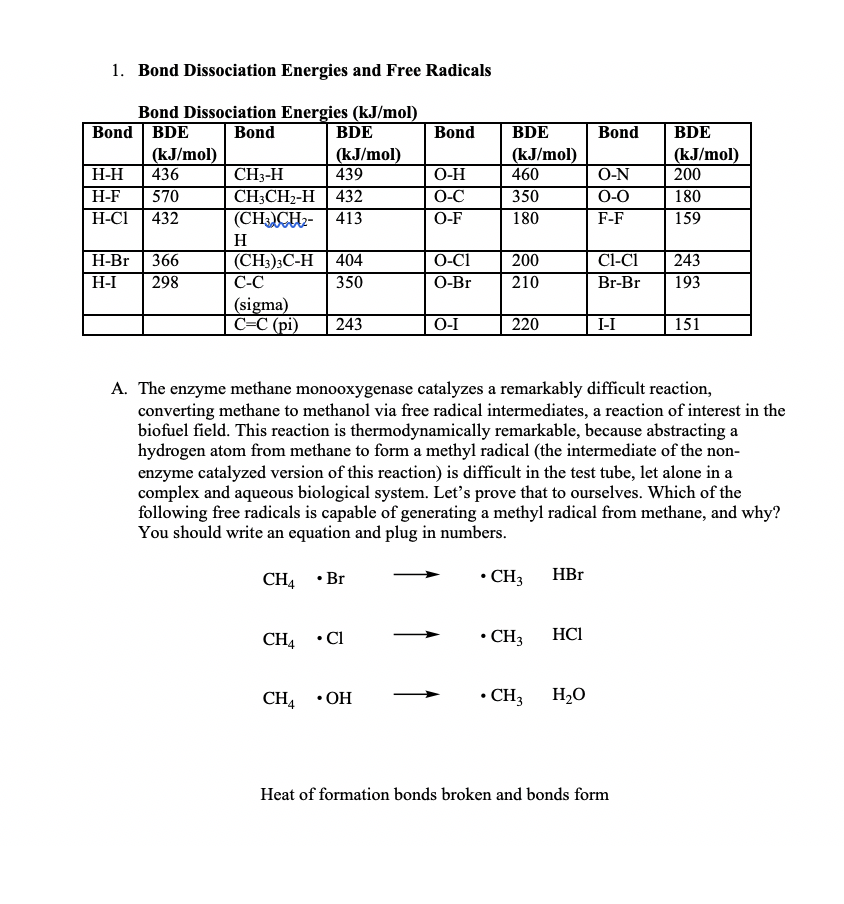1. Bond Dissociation Energies and Free Radicals Bond Bond Dissociation Energies (kJ/mol) Bond BDE Bond BDE (kJ/mol) (kJ/mol) H-H 436 CH3-H 439 H-F 570 CH3CH2-H 432 H-CI 432 (CH3CH2 413 BDE Bond (kJ/mol) 10-N 350 0-0 180 F-F O-H 0-C O-F 460 BDE (kJ/mol) | 200 180 159 | H-Br H-I 366 298 404 350 O-C1200 O-Br (CH3)3C-H C-C (sigma) C=C(pi) 210 C1-C1243 Br-Br 193 243 0 -I 220 I-I | 151 A. The enzyme methane monooxygenase catalyzes a remarkably...

• ### 7. Calculate the AHRxn for the following transformation. A table of bond dissociation energies is provided...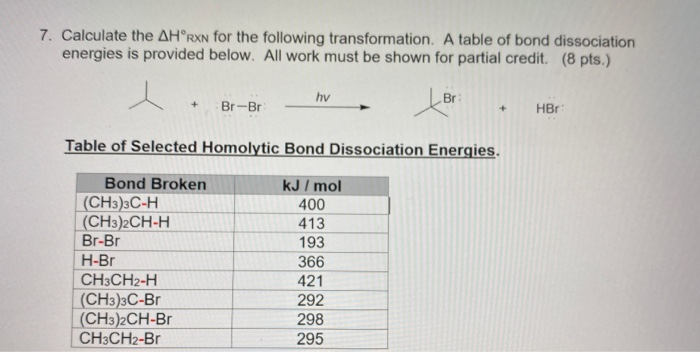7. Calculate the AHRxn for the following transformation. A table of bond dissociation energies is provided below. All work must be shown for partial credit. (8 pts.) hv Br + Br-Br + HBr: Table of Selected Homolytic Bond Dissociation Energies. Bond Broken (CH3)3C-H (CH3)2CH-H Br-Br H-Br CH3CH2-H (CH3)3C-Br (CH3)2CH-Br CH3CH2-Br kJ/mol 400 413 193 366 421 292 298 295

• ### Using the table of bond dissociation enthalpies, calculate AH° for the reaction A. (Use the entries...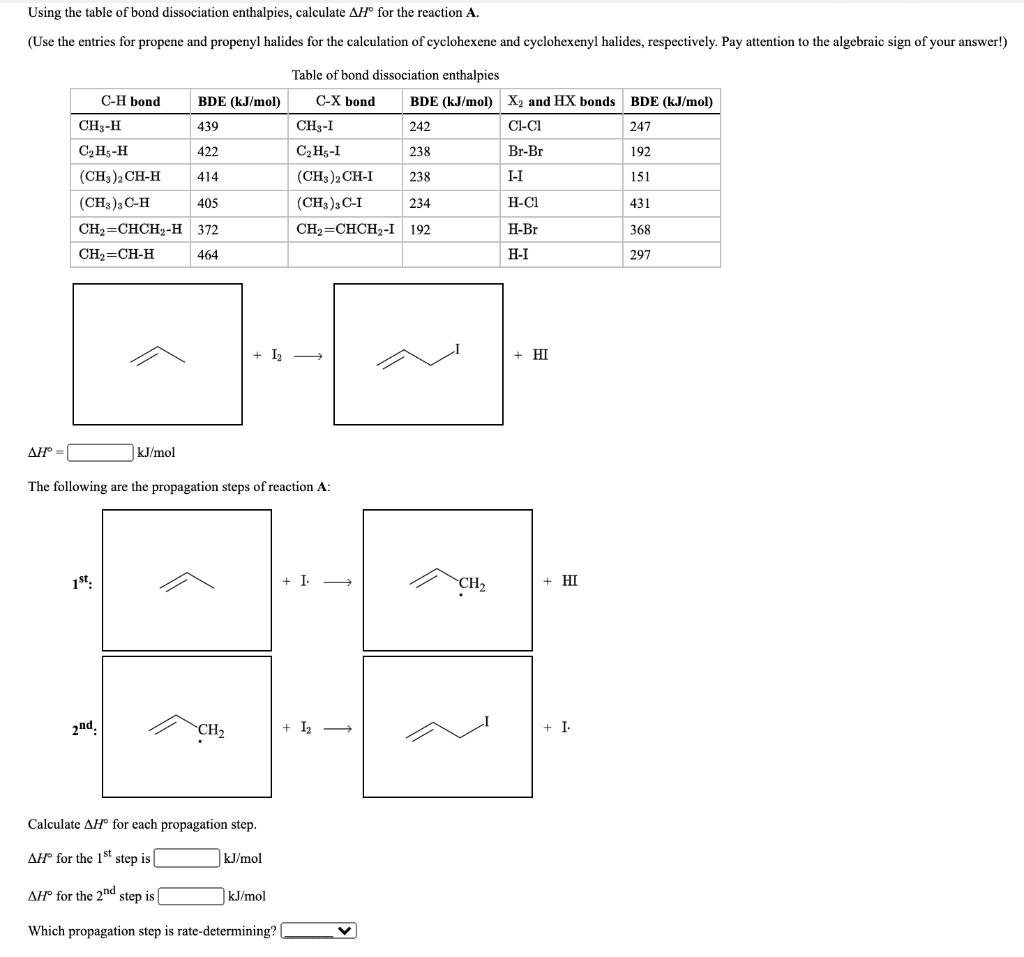Using the table of bond dissociation enthalpies, calculate AH° for the reaction A. (Use the entries for propene and propenyl halides for the calculation of cyclohexene and cyclohexenyl halides, respectively. Pay attention to the algebraic sign of your answer!) C-H bond BDE (kJ/mol) CH3-H 439 C2H:-H 422 (CH3), CH-H 414 (CH3)3C-H 405 CH2=CHCH2-H 372 CH2=CH-H 464 Table of bond dissociation enthalpies C-X bond BDE (kJ/mol) X, and HX bonds BDE (kJ/mol) CH3-I 242 CI-CI 247 C2H5-I 238 Br-Br 192 (CH3)2...

• ### Problem #3 Calculate AH° for the following reaction using the bond energies found in Table 6.1...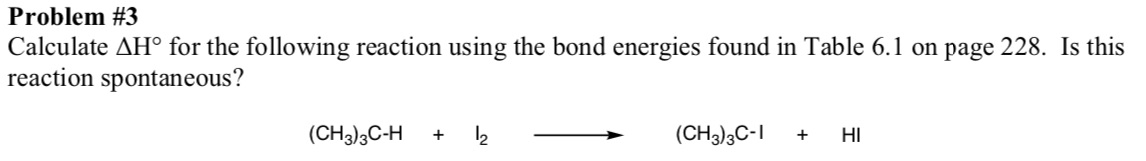Problem #3 Calculate AH° for the following reaction using the bond energies found in Table 6.1 on page 228. Is this reaction spontaneous? (CH3)3C-H + 12 (CH3)3C-1 + HI TABLE 6.1 BOND DISSOCIATION ENERGIES (AH) OF COMMON BONDS KJ/MOL KCAL/MOL H2C=CH-CH3 HC-C-CH KJ/MOL KCAL/MOL 385 92 489117 KJ/MOL KCAL/MOL 444 106 335 80 285 Bonds to H. H-H H-CH3 H-CH2CH3 H-CH(CH3)2 H-C(CH3)3 (CH3)2CH-F (CH3)2CH-CI (CH3)2CH-Br (CH3)2CH- (CH3)2CH-OH 68 222 435 104 435 104 41098 39795 91 473 113 53 381...

• ### H2C=CH2 + CI H2C=CH + HCI Use the table of bond dissociation energies above to calculate...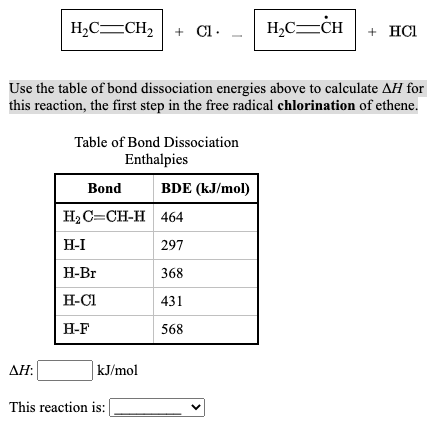H2C=CH2 + CI H2C=CH + HCI Use the table of bond dissociation energies above to calculate AH for this reaction, the first step in the free radical chlorination of ethene. Table of Bond Dissociation Enthalpies Bond BDE (kJ/mol) H2C=CH-H 464 H-I 297 H-Br 368 H-C1 431 H-F 568 ΔΗ: kJ/mol This reaction is:

• ### TABLE 6.1 BOND DISSOCIATION ENERGIES (A) OF COMMON BONDS KJ/MOL KCAL/MOL Bonds to H H2C=CH-CH2 HCC-CH,...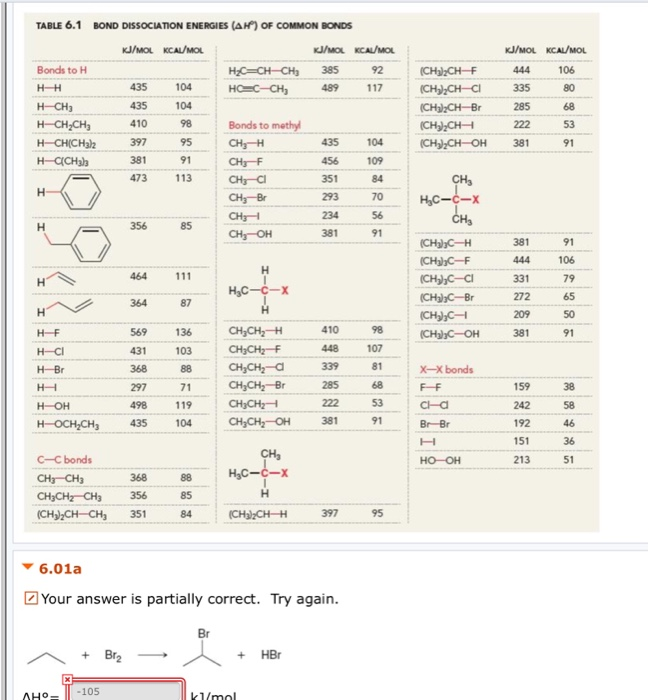TABLE 6.1 BOND DISSOCIATION ENERGIES (A) OF COMMON BONDS KJ/MOL KCAL/MOL Bonds to H H2C=CH-CH2 HCC-CH, KJ/MOL KCAL/MOL 385 92 489117 HH 435 80 104 104 KJ/MOL KCAL/MOL 444 106 335 285 222 381 91 (CH3)2CH-F (CH) CH-CI (CH3)2CHBr (CH),CH (CH3)2CH-OH H-CH3 H-CH2CH3 HCH(CH3 H- CH3) 435 104 109 Bonds to methyl CH; CHEF CH3-C1 CH3-Br CH3 CH, OH CH I I 356 85 381 91 HC-c-X CHE (CH3)3C-H (CH3),C-F (CH),C-C1 (CH3)2C-Br (CH3), (CH)C-OH 381 444 331 106 111 464...

• ### Part A. Given the bond dissociation energies (in kJ/mol) for the following diatomic molecules Cl2 (243),...

Part A. Given the bond dissociation energies (in kJ/mol) for the following diatomic molecules Cl2 (243), F2 (158), H2 (436), O2 (498), N2 (945) choose the one(s) that could be broken by using blue light (λ=465 nm). Part B. Given the bond energies (in kJ/mol) of the following bonds: F–F (155), F–Cl (193), and Cl–Cl (243), estimate the molar enthalpy of formation of ClF(g), that is find ∆H for the following reaction ½Cl2(g) + ½F2(g) → ClF(g)

• ### BO D Which of ten The reaction of A to B. B) The reaction of B...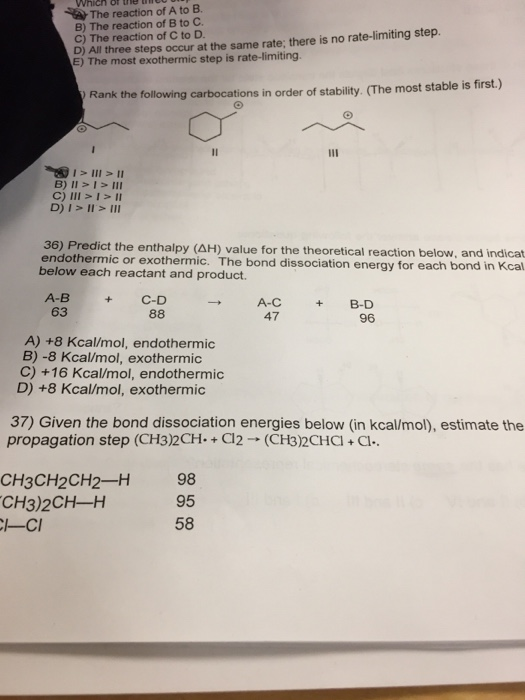BO D Which of ten The reaction of A to B. B) The reaction of B to C. C) The reaction of C to D. D) All three steps occur at the same rate: there is no rate-limiting step. E) The most exothermic step is rate-limiting. esaretim Rank the following carbocations in order of stability. (The most stable is first.) > III II B) II > > III C) III > > II I > Il > III D 36)...

• ### Question 1a. (0.5 point) Consider the bond dissociation energies listed below in kcal/mol CH3 – Br...

Question 1a. (0.5 point) Consider the bond dissociation energies listed below in kcal/mol CH3 – Br 70 CH3CH2 – Br 68 (CH3)2CH – Br 68 (CH3)3C – Br 65 These data show that the carbon–bromine bond is weakest when bromine is bound to a ____. a) methyl carbon b) primary carbon c) secondary carbon d) tertiary carbon e) quaternary carbon 1b. Predict the product for the halogenation reaction given below when methyl cyclohexane is subjected to free radical bromination Br2...